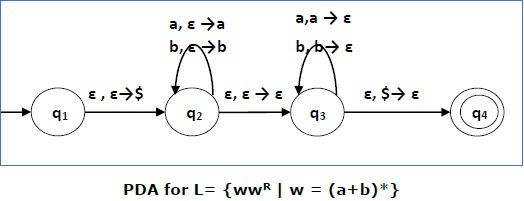# Pushdown Automata Acceptance

There are two different ways to define PDA acceptability.

## Final State Acceptability

In final state acceptability, a PDA accepts a string when, after reading the entire string, the PDA is in a final state. From the starting state, we can make moves that end up in a final state with any stack values. The stack values are irrelevant as long as we end up in a final state.

For a PDA (Q, ∑, S, δ, q0, I, F), the language accepted by the set of final states F is −

L(PDA) = {w | (q0, w, I) ⊢* (q, ε, x), q ∈ F}

for any input stack string x.

## Empty Stack Acceptability

Here a PDA accepts a string when, after reading the entire string, the PDA has emptied its stack.

For a PDA (Q, ∑, S, δ, q0, I, F), the language accepted by the empty stack is −

L(PDA) = {w | (q0, w, I) ⊢* (q, ε, ε), q ∈ Q}

### Example

Construct a PDA that accepts L = {0n 1n | n ≥ 0}

### SolutionThis language accepts L = {ε, 01, 0011, 000111, ............................. }

Here, in this example, the number of ‘a’ and ‘b’ have to be same.

• Initially we put a special symbol ‘\$’ into the empty stack.

• Then at state q2, if we encounter input 0 and top is Null, we push 0 into stack. This may iterate. And if we encounter input 1 and top is 0, we pop this 0.

• Then at state q3, if we encounter input 1 and top is 0, we pop this 0. This may also iterate. And if we encounter input 1 and top is 0, we pop the top element.

• If the special symbol ‘\$’ is encountered at top of the stack, it is popped out and it finally goes to the accepting state q4.

### Example

Construct a PDA that accepts L = { wwR | w = (a+b)* }

SolutionInitially we put a special symbol ‘\$’ into the empty stack. At state q2, the w is being read. In state q3, each 0 or 1 is popped when it matches the input. If any other input is given, the PDA will go to a dead state. When we reach that special symbol ‘\$’, we go to the accepting state q4.